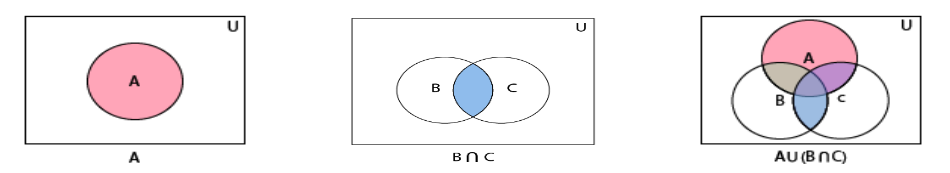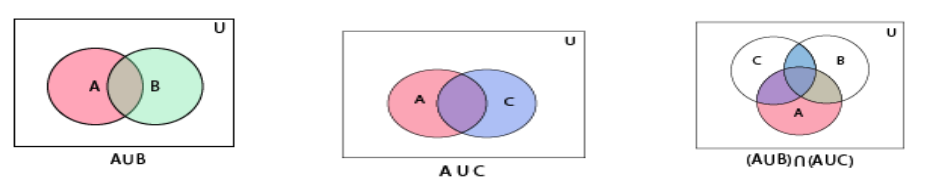Set Theory: A ∪ (B ∩ C) = (A ∪ B) ∩ (A ∪ C)

## Objective

To verify distributive law for three given non-empty sets A, B and C, that is, A ∪ (B ∩ C) = (A ∪ B) ∩ (A ∪ C)

## Distributive Law

• Distributive Law states that, the sum and product remain the same value even when the order of the elements is altered.
• We will see the distributive property of sets using Venn diagram. Before start with the distributive property. Lets see some basic concepts of set theory.
• Distributive laws for three given non-empty sets A, B, and C, that is, A∪(B∩C) = (A∪B)∩(A∪C)

## Venn Diagram

A Venn diagram in math is used in logic theory and set theory to show various sets of data and their relationship with each other.

## Set Theory

• Set Theory is a branch of mathematical logic where we learn sets and their properties. A set is a collection of well defined objects or groups of objects.
• These objects are often called elements or members of a set. For example, a group of players in a cricket team is a set.

## Null Set

In mathematical sets, the null set, also called the empty set, is the set that does not contain anything.

## Non-Empty Set

• A nonempty set is a set containing one or more elements. Any set other than the empty set is called non-empty set. Nonempty sets are sometimes also called nonvoid sets.
• A nonempty set containing a single element is called a singleton set.

## Union

The union of two sets A and B is the set of all those elements which are either in A or B, i.e. A ∪ B

## Intersection

The intersection of two sets A and B is the set of all common elements. The intersection of these two sets is denoted by A∩B.

## Representation of LHS relations of distributive law of set theory## Representation of RHS relations of distributive law of set theory